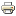# Notation Used in this CoursePrinter-friendly version

Notation used in the course.

• $b_0$ ("b-zero"): estimated sample y-intercept in a linear regression model (more generally, estimated value of $y$ when all the predictors equal zero) [notation for this is $\hat{b}_0$ ("b-zero-hat") in the textbook]
• $\beta_0$ ("beta-zero"): population y-intercept in a regression model [$b_0$ ("b-zero") in the textbook]
• $b_1$ ("b-one"): estimated sample slope in a linear regression model (more generally, estimated sample change in $y$ for a one-unit increase in the corresponding predictor, holding all other predictors constant) [$\hat{b}_1$ ("b-one-hat") in the textbook]
• $\beta_1$ ("beta-one"): population slope in a linear regression model [$b_1$ ("b-one") in the textbook]
• $e_i$: i-th (sample) prediction error (or residual error), equal to $y_i-\hat{y}_i$ [$\hat{e}_i$ in the textbook]
• $\epsilon_i$ ("epsilon-i"): i-th (population) error, equal to $y_i-\mbox{E}(Y_i)$ [$e_i$ in the textbook]
• $i$: index for the i-th obeservation or experimental unit
• $n$: sample size (total number of observations)
• $k$: number of predictor terms in a linear regression model, which means there are $k+1$ regression coefficients (including the intercept).
• $r$: (Pearson) correlation coefficient between two quantitative variables
• $r^2$ ("r-squared"): coefficient of determination in a simple linear regression model, equal to $SSR$/$SSTO$
• $R^2$ ("R-squared"): coefficient of determination in a multiple linear regression model, equal to $SSR$/$SSTO$
• $SSR$: regression sum of squares (measures deviations of $\hat{y}$ from $\bar{y}$)
• $SSE$: error sum of squares (measures deviations of $y$ from $\hat{y}$) [$RSS$ (residual sum of squares) in the textbook]
• $SSTO$: total sum of squares (measures deviations of $y$ from $\bar{y}$) [$TSS$ in the textbook]
• $MSE$ ("mean square error"): (sample) mean square prediction error (or residual error)
• $s$: regression (residual) standard error (square root of MSE)
• $\sigma^2$ ("sigma-squared"): (population) common error variance in a linear regression model
• $x$: a predictor, explanatory, or independent variable in a linear regression model
• $\bar{x}$ ("x-bar"): sample mean of $x$
• $y$: the response, outcome, or dependent variable in a linear regression model
• $\bar{y}$ ("y-bar"): (univariate) sample mean of $y$ (ignoring any predictors)
• $\hat{y}$ ("y-hat"): predicted or fitted value of $y$ in a linear regression model (i.e., accounting for the predictors)
• $\mbox{E}(Y)$ or $\mu_Y$ ("expected value of Y"): population mean of Y in a linear regression model